What is the meaning of Sigma Symbol?

Greek Alphabet Sigma has been used widely as a notation for summationIndex is always integer.

LastIndex must be greater or equal to the firstIndex .

Example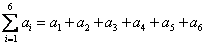If we have a sequence of numbers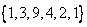then we can put index on the sequence such that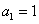,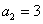,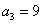,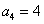,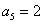,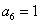and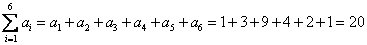Why do we need to put a sigma symbol?

Using this symbol, you can simplify the notation of addition. Instead of writing all the symbols such as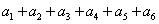, you can type them neatly as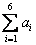. Aside from that, using the sigma notation, you may manipulate the sums algebraically without even need to expand them all the sums. Check later in the summation tricks and properties on this tutorial on how to do such algebra manipulation.

Sometimes I see the sigma symbol is without index, what is the meaning?

When the range of index is clear, the firstIndex and lastIndex can be omitted. You do not need to write from where it begins and until what index it stops if people can already understand without them.

Example:

MatrixTrace of a matrix is the summation of diagonal element of a matrix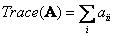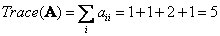When the index is clear, even the index name can be omitted. You do not need to put any index at all. The notation becomes even neater.

Example : Vector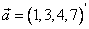Length of vector is a square root of the sum of square of vector component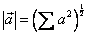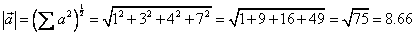< Previous | Next | Contents >